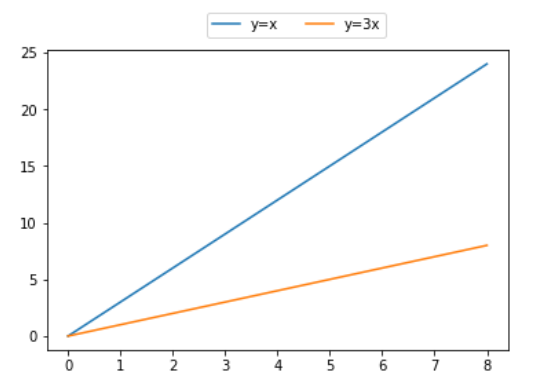Open In App
Related Articles
• Write an Interview Experience

# Matplotlib.pyplot.legend() in Python

Matplotlib is one of the most popular Python packages used for data visualization. It is a cross-platform library for making 2D plots from data in arrays. Pyplot is a collection of command style functions that make matplotlib work like MATLAB. Each pyplot function makes some change to a figure: e.g., creates a figure, creates a plotting area in a figure, plots some lines in a plotting area, decorates the plot with labels, etc.

## Matplotlib.pyplot.legend()

A legend is an area describing the elements of the graph. In the matplotlib library, there’s a function called legend() which is used to Place a legend on the axes.

The attribute Loc in `legend()` is used to specify the location of the legend.Default value of loc is loc=”best” (upper left). The strings ‘upper left’, ‘upper right’, ‘lower left’, ‘lower right’ place the legend at the corresponding corner of the axes/figure.

The attribute bbox_to_anchor=(x, y) of legend() function is used to specify the coordinates of the legend, and the attribute ncol represents the number of columns that the legend has.It’s default value is 1.

Syntax:

matplotlib.pyplot.legend([“blue”, “green”], bbox_to_anchor=(0.75, 1.15), ncol=2)

The Following are some more attributes of function `legend()` :

• shadow: [None or bool] Whether to draw a shadow behind the legend.It’s Default value is None.
• markerscale: [None or int or float] The relative size of legend markers compared with the originally drawn ones.The Default is None.
• numpoints: [None or int] The number of marker points in the legend when creating a legend entry for a Line2D (line).The Default is None.
• fontsize: The font size of the legend.If the value is numeric the size will be the absolute font size in points.
• facecolor: [None or “inherit” or color] The legend’s background color.
• edgecolor: [None or “inherit” or color] The legend’s background patch edge color.

#### Ways to use legend() function in Python –

Example 1:

 `import` `numpy as np``import` `matplotlib.pyplot as plt`` ` `# X-axis values``x ``=` `[``1``, ``2``, ``3``, ``4``, ``5``]`` ` `# Y-axis values ``y ``=` `[``1``, ``4``, ``9``, ``16``, ``25``]`` ` `# Function to plot  ``plt.plot(x, y)`` ` `# Function add a legend  ``plt.legend([``'single element'``])`` ` `# function to show the plot``plt.show()`

Output :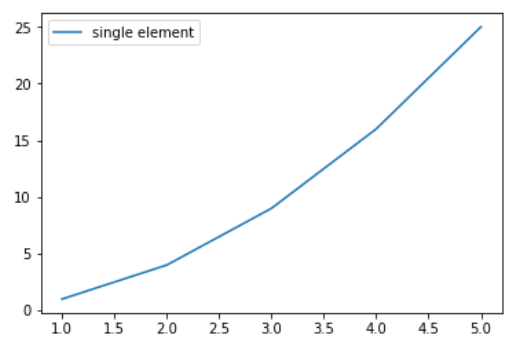Example 2:

 `# importing modules``import` `numpy as np``import` `matplotlib.pyplot as plt`` ` `# Y-axis values``y1 ``=` `[``2``, ``3``, ``4.5``]`` ` `# Y-axis values ``y2 ``=` `[``1``, ``1.5``, ``5``]`` ` `# Function to plot  ``plt.plot(y1)``plt.plot(y2)`` ` `# Function add a legend  ``plt.legend([``"blue"``, ``"green"``], loc ``=``"lower right"``)`` ` `# function to show the plot``plt.show()`

Output :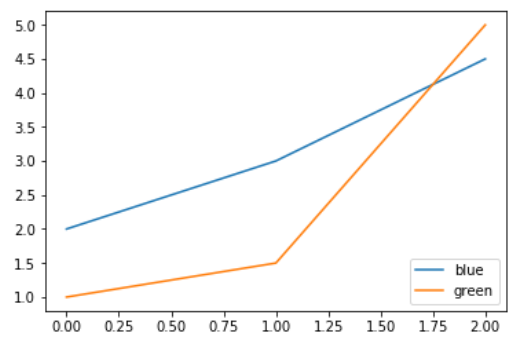Example 3:

 `import` `numpy as np``import` `matplotlib.pyplot as plt`` ` `# X-axis values``x ``=` `np.arange(``5``)`` ` `# Y-axis values``y1 ``=` `[``1``, ``2``, ``3``, ``4``, ``5``]`` ` `# Y-axis values ``y2 ``=` `[``1``, ``4``, ``9``, ``16``, ``25``]`` ` `# Function to plot  ``plt.plot(x, y1, label ``=``'Numbers'``)``plt.plot(x, y2, label ``=``'Square of numbers'``)`` ` `# Function add a legend  ``plt.legend()`` ` `# function to show the plot``plt.show()`

Output :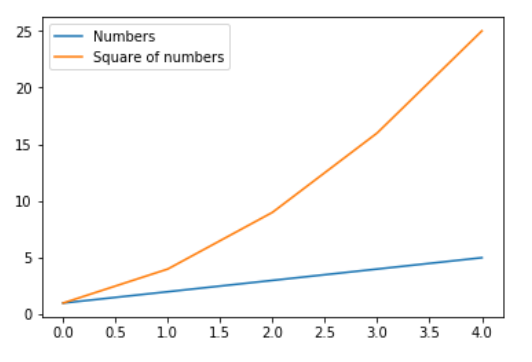Example 4:

 `import` `numpy as np``import` `matplotlib.pyplot as plt`` ` `x ``=` `np.linspace(``0``, ``10``, ``1000``)``fig, ax ``=` `plt.subplots()`` ` `ax.plot(x, np.sin(x), ``'--b'``, label ``=``'Sine'``)``ax.plot(x, np.cos(x), c ``=``'r'``, label ``=``'Cosine'``)``ax.axis(``'equal'``)`` ` `leg ``=` `ax.legend(loc ``=``"lower left"``);`

Output: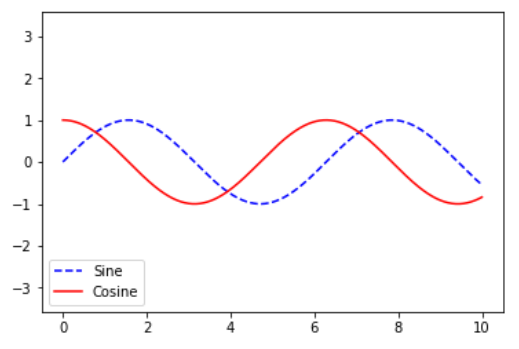Example 5:

 `# importing modules``import` `numpy as np``import` `matplotlib.pyplot as plt``  ` `# X-axis values``x ``=` `[``0``, ``1``, ``2``, ``3``, ``4``, ``5``, ``6``, ``7``, ``8``]``  ` `# Y-axis values``y1 ``=` `[``0``, ``3``, ``6``, ``9``, ``12``, ``15``, ``18``, ``21``, ``24``]``# Y-axis values ``y2 ``=` `[``0``, ``1``, ``2``, ``3``, ``4``, ``5``, ``6``, ``7``, ``8``]``  ` `# Function to plot  ``plt.plot(y1, label ``=``"y = x"``)``plt.plot(y2, label ``=``"y = 3x"``)``  ` `# Function add a legend  ``plt.legend(bbox_to_anchor ``=``(``0.75``, ``1.15``), ncol ``=` `2``)``  ` `# function to show the plot``plt.show()`

Output: